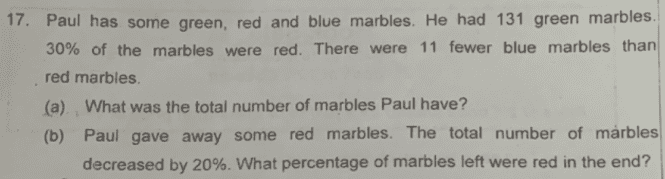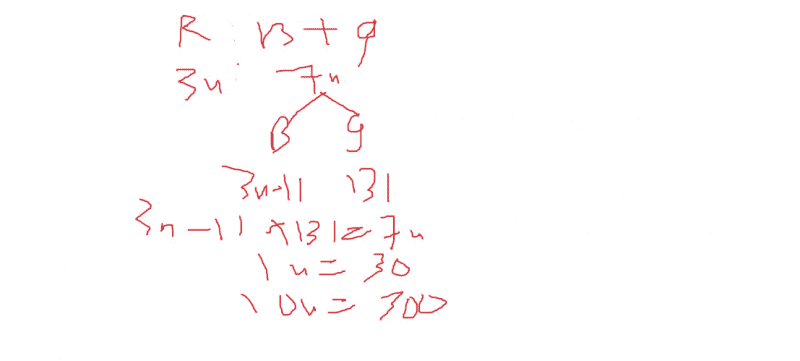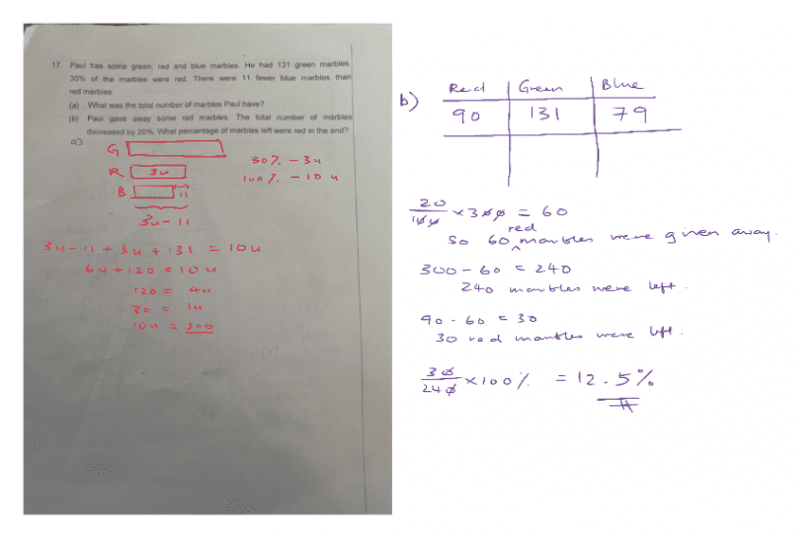# Questionusing ratio branching out method

part b should be straight forward for most

0 Replies 1 Like

Thanks @SavingPrivateTutor!  Here’s my solution using just equations.

a.
131 + (30/100)T + (30/100)T – 11 = T
131 + 60/100T – 11 = T
40/100T = 120
=> T = (100/40)120 = 300 #

b.
Number of marbles at first:
Red = 300*30/100 = 90
Green = 131
Blue = 90 – 11 = 79

When he gave away the Red marbles, total number of marbles decreased by 20/100*300 = 60
So, number of Red marbles remaining = 90 – 60 = 30
So, % of Red marbles in the end = 30/(300 – 60) x 100 = 12.5% #

0 Replies 3 Likes